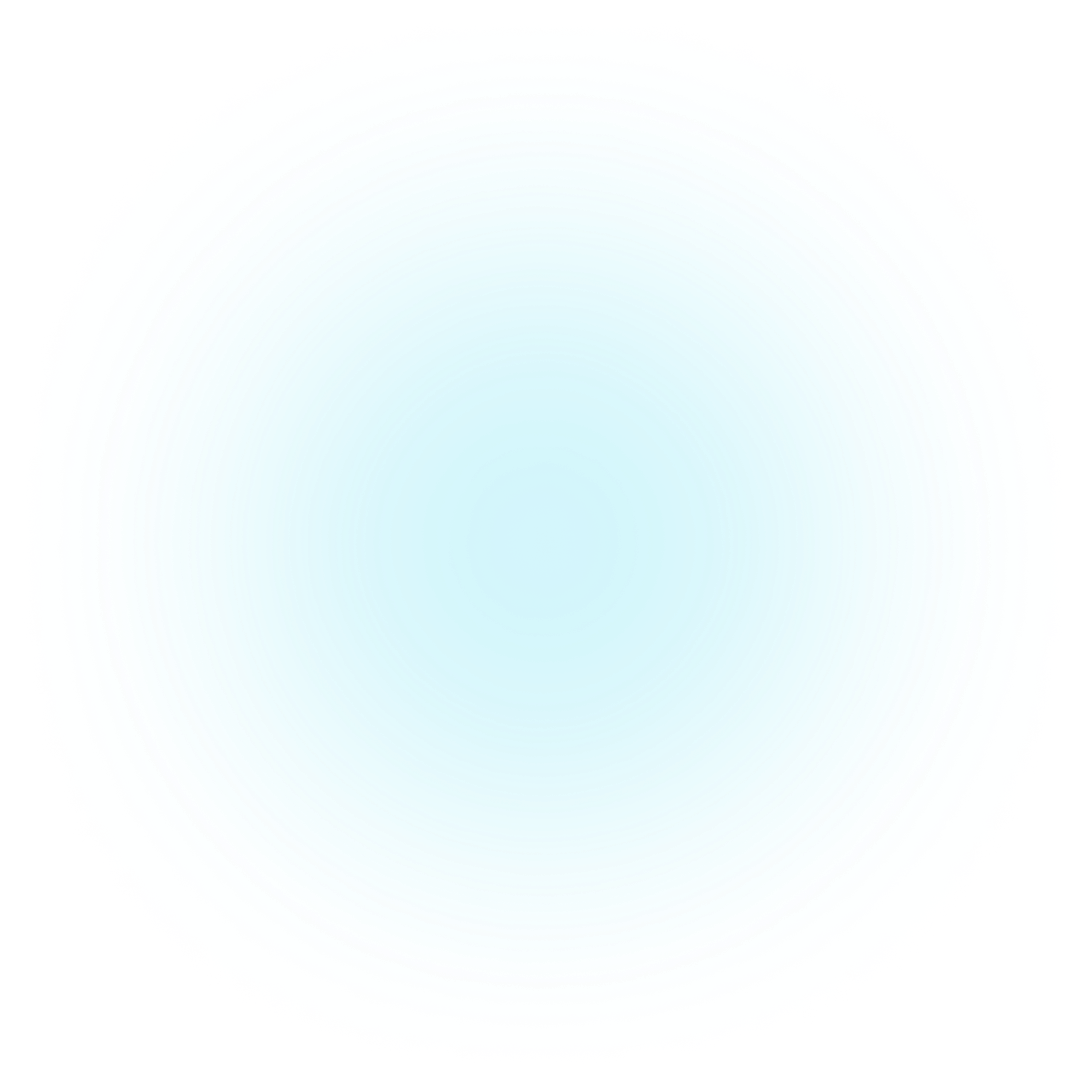Easily turn your data into engaging visualizations. Powerful API for coders. Powerful app for everyone.main.py
notebook.ipynb
main.rs
``````from plotapi import Chord

Chord(matrix, names).show()``````

Visualizations Showcase

# Pokemon Types with Heat Map

In this notebook we're going to use PlotAPI Heat Map to visualise the co-occurrences between Pokémon types. We"ll use Python, but PlotAPI can be used from any programming language.

## Preamble

``````import json

from plotapi import HeatMap
``````

## Introduction

In this notebook we're going to use PlotAPI HeatMap to visualise the co-occurrences between Pokémon types. We"ll use Python, but PlotAPI can be used from any programming language.

## Dataset

We're going to use Pokémon (Gen 1-8) data, a fork of which is available in this repository. Let"s get loading the data.

``````with open("pokemon_types.json", "r") as f:

names = ["Bug", "Dark", "Dragon", "Electric", "Fairy", "Fighting",
"Fire", "Flying", "Ghost", "Grass", "Ground", "Ice",
"Normal", "Poison", "Psychic", "Rock", "Steel", "Water"]
``````

## Visualisation

Let's use PlotAPI Chord for this visualisation.

We're going to adjust some layout and template parameters, and flip the intro animation on too.

Because we're using a data-table, we can also click on any part of the diagram to "lock" the selection.

``````plot = HeatMap(
data["matrix"],
col_names=names,
row_names=names,
colors="turbo",
details_thumbs=data["details_thumbs"],
noun="Pokemon!",
thumbs_width=50,
thumbs_margin=1,
popup_width=600,
data_table_column_width=100,
data_table=data["data_table"],
data_table_show_indices=False,
zero_color="transparent",
scale="exponential",
scale_exponent=0.40,
)
``````

### Display inline

``````plot
``````

With our plot created, let's upload it to PlotAPI cloud and get a shareable link.

``````plot.upload(
name="Pokémon Types (Gen 1-9)",
description='''In this project we're going to use PlotAPI to visualize the co-occurrences between Pokémon types. We're going to use Pokémon (Gen 1-9) data, a fork of which is available in @shahinrostami's GitHub.''',
maximized=True,
full_width=True,
public=True,
custom_css=custom_css
)
``````
Your visualization has been uploaded successfully! You can view and share it at https://plotapi.com/explore/view/fb28b635-bbb2-4f6c-b75d-56e9ba2b10f0.
Previous
Showcase